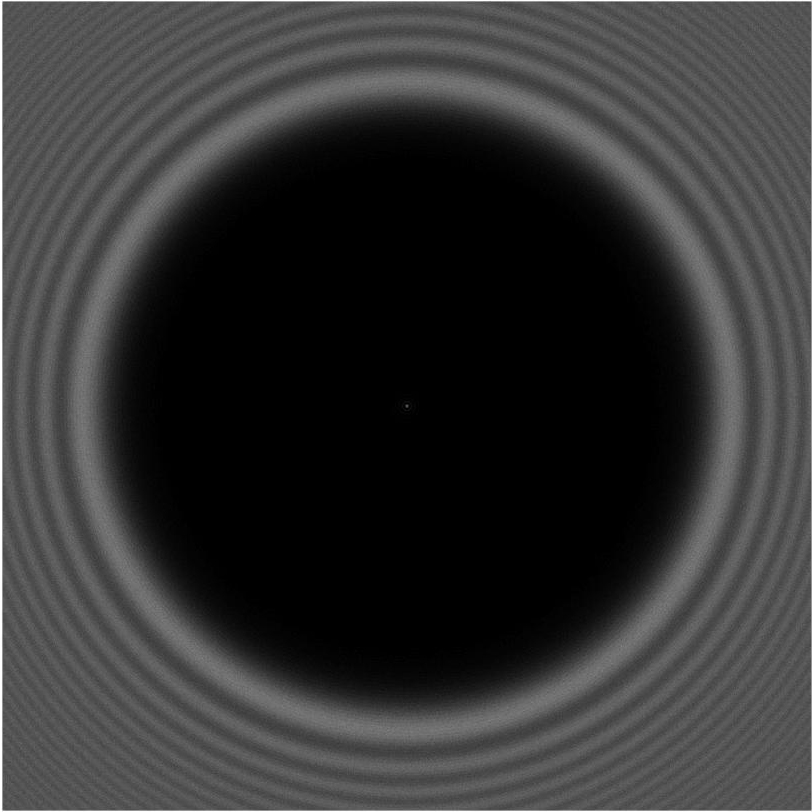## Poisson's Spot (aka Spot of Arago)

Occulter/aperture size is $$\sf D = 60$$mm.
Wavelength is $$\sf \lambda = 600$$nm.
Downstream distance starts at $$\sf z = 25 D = 1.5$$m and tends toward infinity.

The formula for the downstream electromagnetic field associated with a plane wave after passing through a circular opening measured as a function of radius $$\sf \rho$$ from the beam/shadow's center is:

$$\sf E_{hole}(\rho) = \frac{2 \pi}{i \lambda z} e^{\frac{i \pi \rho^2 }{ \lambda z}} \int_0^{D/2} J_0(2\pi \rho r / \lambda z) e^{\frac{i \pi r^2 }{ \lambda z}} r dr$$

By Babinet's principle, the downstream electric field associated with a circular occulter is just one minus the above electric field:

$$\sf E_{occulter}(\rho) = 1 - E_{hole}(\rho)$$

The intensity (aka brightness) of the image is the magnitude-squared of the complex electromagnetic field. The movies below show the brightness.

In the 2D animations shown on the left below, each pixel in each frame is a realization of a Poisson random variable having a mean value proportional to the current intensity at that location.
These values represent the number of discrete photons arriving during an "exposure".

The movie starts out slow but has a dramatic ending---hit the "play" button.

Here's a closeup showing Poisson's spot at the center...